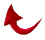Awesome Mathematical Miracle Confirms the Number of A.L.M.’s in Suras 2 and 3

By Dr. Ali R. Fazely

God’s mathematical miracle of the Quran is destined to overwhelm the whole world and prove beyond a shadow of a doubt that the Quran is the unaltered word of the Almighty, God.  Here, based on advanced mathematics of primes and their indices which is yet simple to understand, we see that the counts reported by Dr. Rashad Khalifa were in fact the true number of A.L.M.’s in Suras 2 and 3 and divinely inspired by God to His Messenger of the Covenant.

We know that the number of letters in the first verse in the Quran is the prime number 19.  We also know that the index of prime number 19 is 8, or in other words the 8th prime number is 19.  If I multiply 8 by 19, I get 152.  Recall that the four words in the opening verse “NAME”, “GOD”, “MOST GRACIOUS”, AND “MOST MERCIFUL” are repeated throughout the Quran and are exact multiples of 19.  More precisely, these four words are repeated 19, 2698, 57, and 114 times, respectively.  These are shown below

 19 = 19 x 1   2698 = 19 x 142   57 = 19 x 3   114 = 19 x 6

152 = 8 x 19

This was first reported by Dr. Khalifa in his book, “The Visual Presentation of the Miracle”.  Note here, that the importance of 152 is that it is not just any multiple of 19, but it is a product of 19 and its index 8.

Now let us look at the number 152.  There are three digits that make up this number, namely 1, 2, and 5.   Out of these three digits we can make 6 different 3 digit numbers.  These are:

152      125      215      251      512      521

These numbers are called the “permutations” of 1, 2, and 5.  Note here that only two of these permutations are primes.  I tabulated these two prime numbers together with their indices as shown below:

 Prime Index 251 54 521 98 152 = 8 x 19

19 x 521 = 9899

This is the number of A.L.M.’s in Sura 2, Glory be to God.  Now if I concatenate 2 and 98, I get 298, and again get ready for this!

19 x 298 = 5662

This is the number of A.L.M.’s in sura 3!  The relation between Sura 2 and Sura 3 is that prime number 2 is the index of prime number 3.

Now let us examine 521 and 251 and their indices more closely.  In Sura 54 called, “The Moon”, God talks about the signs we should watch for when the end of the world is near.  In the first verse the splitting of the moon is mentioned.  Verse 54:2 speaks of the Mathematical Miracle of the Quran,  Then they saw a miracle; but they turned away and said, ‘Old magic.”  “In Sura 98 entitled, “The Proof” God again refers to the Mathematial Miracle of the Quran.  Now if I add 521 and 98, I get:

521 + 98 = 619

Which is a prime number and it is the “114th” Prime Number.  Glory be to God!  It is noteworthy that the gematrical value of Rashad, 505 and 114 yield:

505 + 114 = 619 (which is the 114th prime)

In Sura 3 we have 298 and 5662 to study.  Note here that:

2 + 9 + 8 = 19

and,

5 + 6 +6 + 2 = 19

If I add the coefficients of the number 19 in both Suras I get:

521 + 298 = 819

This is again a combination of 8 and 19, or 19 and its index 8.  Indeed the Mathematical Miracle of the Quran is ONE OF THE GREAT MIRACLES (74:35).

Therefore, we can see that advanced mathematics is involved here and is beyond the capabilities of humans and jinns together armed with all the assistance they could give each other.  Therefore, these coefficients, by God’s design, must be 521 and 298 not just any meaningless number multiplied by 19!

All praises are due to God, Creator of all things.Return to Table of Contents

/